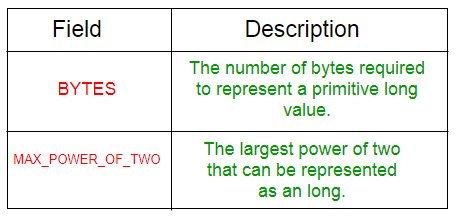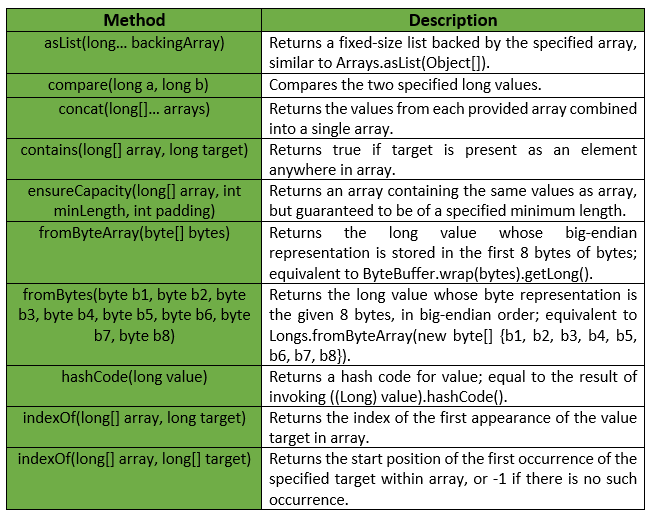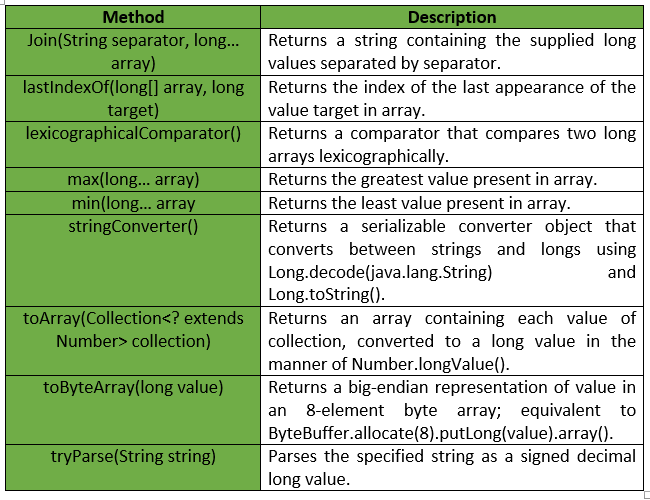# Longs Class | Guava | Java

Longs is a utility class for primitive type long. It provides Static utility methods pertaining to long primitives, that are not already found in either Long or Arrays.

Declaration :

```@GwtCompatible(emulated=true)
public final class Longs
extends Object
```

Below table shows the Field summary for Guava Longs Class :Some of the methods provided by Guava Longs Class are :Exceptions :

• min : IllegalArgumentException if array is empty.
• max : IllegalArgumentException if array is empty.
• fromByteArray : IllegalArgumentException if bytes has fewer than 8 elements.
• ensureCapacity : IllegalArgumentException if minLength or padding is negative.
• toArray : NullPointerException if collection or any of its elements is null.

Below table shows some other methods provided by Guava Longs Class :Below given are some examples showing the implementation of methods of Guava Longs Class :
Example 1 :

 `// Java code to show implementation ` `// of Guava Longs.asList() method ` ` `  `import` `com.google.common.primitives.Longs; ` `import` `java.util.*; ` ` `  `class` `GFG { ` `    ``// Driver method ` `    ``public` `static` `void` `main(String[] args) ` `    ``{ ` `        ``long` `arr[] = { 3L, 4L, 5L, 6L, 7L }; ` ` `  `        ``// Using Longs.asList() method which wraps ` `        ``// the primitive long array as List of ` `        ``// long Type ` `        ``List myList = Longs.asList(arr); ` ` `  `        ``// Displaying the elements ` `        ``System.out.println(myList); ` `    ``} ` `} `

Output :

```[3, 4, 5, 6, 7]
```

Example 2 :

 `// Java code to show implementation ` `// of Guava Longs.toArray() method ` ` `  `import` `com.google.common.primitives.Longs; ` `import` `java.util.*; ` ` `  `class` `GFG { ` `    ``// Driver method ` `    ``public` `static` `void` `main(String[] args) ` `    ``{ ` `        ``List myList = Arrays.asList(3L, 4L, 5L, 6L, 7L); ` ` `  `        ``// Using Longs.toArray() method which ` `        ``// converts a List of Longs to an ` `        ``// array of long ` `        ``long``[] arr = Longs.toArray(myList); ` ` `  `        ``// Displaying the elements ` `        ``System.out.println(Arrays.toString(arr)); ` `    ``} ` `} `

Output :

```[3, 4, 5, 6, 7]
```

Example 3 :

 `// Java code to show implementation ` `// of Guava Longs.concat() method ` ` `  `import` `com.google.common.primitives.Longs; ` `import` `java.util.*; ` ` `  `class` `GFG { ` `    ``// Driver method ` `    ``public` `static` `void` `main(String[] args) ` `    ``{ ` `        ``long``[] arr1 = { 3L, 4L, 5L }; ` `        ``long``[] arr2 = { 6L, 7L }; ` ` `  `        ``// Using Longs.concat() method which ` `        ``// combines arrays from specified ` `        ``// arrays into a single array ` `        ``long``[] arr = Longs.concat(arr1, arr2); ` ` `  `        ``// Displaying the elements ` `        ``System.out.println(Arrays.toString(arr)); ` `    ``} ` `} `

Output :

```[3, 4, 5, 6, 7]
```

Example 4 :

 `// Java code to show implementation ` `// of Guava Longs.contains() method ` ` `  `import` `com.google.common.primitives.Longs; ` ` `  `class` `GFG { ` `    ``// Driver method ` `    ``public` `static` `void` `main(String[] args) ` `    ``{ ` `        ``long``[] arr = { 3L, 4L, 5L, 6L }; ` ` `  `        ``// Using Longs.contains() method which ` `        ``// checks if element is present in array ` `        ``// or not ` `        ``System.out.println(Longs.contains(arr, 4L)); ` `        ``System.out.println(Longs.contains(arr, 7L)); ` `    ``} ` `} `

output :

```true
false
```

Example 5 :

 `// Java code to show implementation ` `// of Guava Longs.min() method ` ` `  `import` `com.google.common.primitives.Longs; ` ` `  `class` `GFG { ` `    ``// Driver method ` `    ``public` `static` `void` `main(String[] args) ` `    ``{ ` `        ``long``[] arr = { 3L, 4L, 5L, 6L }; ` ` `  `        ``// Using Longs.min() method ` `        ``System.out.println(Longs.min(arr)); ` `    ``} ` `} `

Output :

```3
```

Example 6 :

 `// Java code to show implementation ` `// of Guava Longs.max() method ` ` `  `import` `com.google.common.primitives.Longs; ` ` `  `class` `GFG { ` `    ``// Driver method ` `    ``public` `static` `void` `main(String[] args) ` `    ``{ ` `        ``long``[] arr = { 3L, 4L, 5L, 6L }; ` ` `  `        ``// Using Longs.max() method ` `        ``System.out.println(Longs.max(arr)); ` `    ``} ` `} `

Output :

```6
```

My Personal Notes arrow_drop_upCheck out this Author's contributed articles.

If you like GeeksforGeeks and would like to contribute, you can also write an article using contribute.geeksforgeeks.org or mail your article to contribute@geeksforgeeks.org. See your article appearing on the GeeksforGeeks main page and help other Geeks.

Please Improve this article if you find anything incorrect by clicking on the "Improve Article" button below.

Article Tags :
Practice Tags :

Be the First to upvote.

Please write to us at contribute@geeksforgeeks.org to report any issue with the above content.